# Control Flow

Let’s study some of the many ways we can wire together expressions, statements (and even declarations) to form useful systems.

## Kinds of Control Flow

Programming languages allow us to express the way that execution components (statements, expressions, and declarations) are wired together to effect a computation.

There are (at least) seven types of flow:

1. Sequencing (do this THEN this THEN this ...)
2. Selection (if, unless, switch, case, ...)
3. Iteration (for, while, repeat, until, ...)
4. Procedural Abstraction (subroutine call)
5. Recursion (you know what this is)
6. Nondeterminacy (do this OR this OR ...that is, choose one arbitrarily or randomly)
7. Concurrency (do multiple things at the same time)

## Execution Components

The three important components are:

• Statements, which are are executed
• Expressions, which are are evaluated
• Declarations, which are are elaborated

In some languages, for example, C++, all of them are rolled into statements, so there is a declaration statement and an expression statement.

```void f() {
cout << "hello";       // expression
int x = 10;            // declaration
if (x < 2) return;     // statement
}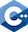```

Some languages don’t have statements at all—code is made up of entirely of expressions. These are called expression-oriented languages. They have while-expressions, not while-statements. Here’s an example in ML:

```- val x = ref 5;
> val x = ref 5 : int ref
- val y = while !x > 0 do (print(Int.toString(!x)^"\n"); x := !x - 1);
5
4
3
2
1
> val y = () : unit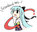```

... and in Ruby:

```>> x = 5
=> 5
>> y = (while x > 0; puts x; x -= 1; end)
5
4
3
2
1
=> nil
>> y
=> nil```

...and in Scala:

```scala> var x = 5
x: Int = 5

scala> var y = (while (x > 0) {println(x); x -= 1;})
5
4
3
2
1
y: Unit = ()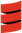```

... and in Algol68:

```begin
a := if b < c then d else e;
a := begin f(b); g(c) end;
g(d);
2 + 3
end             # whole expression evaluates to 5 #
```

## Sequencing

Sequencing is the most basic control flow... it refers to doing elaborations, evaluations, or executions one after another. Not in parallel and not out of order (unless a compiler can guarantee that such optimizations don’t change the meaning).

C uses semi-colons for sequentializing statements and declarations, but uses the comma operator for expressions, for example:

```x = 5;
y = x + 3;                /* x is assigned 5 THEN y is assigned 8 */

x = f(a), g(b), 3;        /* f is called THEN g is called THEN x is assigned 3 */

x = 1,000;                /* x is assigned 0 */```

Standard ML doesn’t have statements, but it can sequence expression evaluation. It uses the semi-colon for sequencing:

```val x = 5;
val y = x                 (* x and y now both 5 *)

val x = (f(a); g(b); 3)   (* x now 3 -- note parens required BTW *)

(* However "and" does parallel binding, NOT sequential *)
val x = y
and y = x                 (* Yes, it’s a swap *)```

You do not always need a sequencing operator; you can get sequencing when calling functions. Here’s some Haskell:

```(\x -> (\y -> "x is " ++ show x ++ " and y is " ++ show y)(x + 3))(5)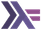```

That gets hard to read, so you’ll often see syntactic sugar such as:

```let x = 5 in
let y = x + 3 in
"x is " ++ show x ++ " and y is " ++ show y```

So, yes, `let`-expressions sugar function calls. Who knew?

## Selection

Selection means we do one of several alternatives. Often done with an "if" statement, for example:

```// Java, C, C++, JavaScript - curly brace languages
if (e1) {
s1
} else if (e2) {
s2
} else if (e3) {
s3
} else if (e4) {
s4
} else {
s5
}
/* For PHP, replace "else if" with "elseif" above */
/* For Perl, replace "else if" with "elsif" above */
```
```# Ruby - terminal end
if e1
s1
elsif e2
s2
elsif e3
s3
elsif e4
s4
else
s5
end```
```# Python - indentation
if e1:
s1
elif e2:
s2
elif e3:
s3
elif e4:
s4
else:
s5```
```# Bash
if e1; then
s1
elif e2; then
s2
elif e3; then
s3
elif e4; then
s4
else
s5
fi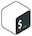```
Exercise: Show the equivalent of the above in Ada, Fortran, Scala, and ML.

Some languages have a syntax that manages to avoid all those “else-ifs”:

```if
e1 -> s1;
e2 -> s2;
e3 -> s3;
e4 -> s4;
true -> s5;
end.```
```; Common Lisp
(cond
((e1) (s1))
((e2) (s2))
((e3) (s3))
((e4) (s4))
(t (s5)))```
```-- Ada
case e is
when c1 => s1;
when c2 => s2;
when c3 => s3;
when c4 => s4;
when others => s5;
end case;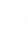```
```// Java
switch (e) {
c1 -> s1;
c2 -> s2;
c3 -> s3;
c4 -> s4;
default -> s5;
}```

Ada even checks to make sure at compile time that the cases are exhaustive!

WARNING WARNING WARNING. Some languages bastardize the conditional so that once you found a truthy condition, you “fall through” and execute subsequent cases:

```// Java, C, JavaScript, C++
switch (e) {
case c1 : s1;    // These "fall through", so you
case c2 : s2;    // ...will normally place a "break"
case c3 : s3;    // ...or a "return" after each
case c4 : s4;
default : s5;    // NOTE: IN JAVA, USE "->"" NOT the ":"
}                    // JAVA -> DOES NOT FALL THROUGH
```

In Go, you don’t fall through, but you can put in a `fallthrough` statement:

```// Go
switch prizeLevel {
case 4:
fmt.Println("Car")
fallthrough
case 3:
fmt.Println("Tent")
fallthrough
case 2:
fmt.Println("T-shirt")
fallthrough
case 1:
fmt.Println("Bandana")
}```
Exercise: Look up the equivalents in lots of other languages. Find out whether they fall through or not, or whether a compiler can check for exhaustive matches.
Fallthrough?

Seems like nuts, right? Well, back in the old days, people found clever ways to use switches with fallthroughs. If you are looking for a fun little history project, research it!

In our modern era, readability is in and cleverness is out (and machines are really fast), so it might be good to follow this advice: Avoid switches in languages with implicit fall-through.

Perl and Ruby have an `unless` statement

```# Perl
unless (\$x >= 0) {   # Braces required in Perl for compound statements
die "must be non-negative";
}```

Perl and Ruby allow if and unless to be used as modifiers for simple statements — allowing you to dispense with the braces or "end"s

```# Perl
die "must be non-negative" unless \$x >= 0;
die "must be non-negative" if \$x < 0;        # Same as above```

Finally short-circuit operators can be used for selection too...

```# Perl
chdir \$dir or die "Can’t change to \$dir: \$!";
open F, \$file or die "Can’t open \$file: \$!";
@lines = <F> or die "\$file may be empty";
close F or die "Can’t close \$file: \$!";```

This is appealing code because one’s eye can scan the "normal" flow of control down the left margin.

Exercise: Write the above using (ugly) if-statements.
Exercise: Clojure has a really interesting take on its `cond`. Check it out. What is different about it?

## Iteration

Iteration is executing code repeatedly, either for each value in an enumeration, or while some condition holds. Iteration is normally modeled by a loop construct, or an iterator object, or by functions with names like `forEach`.

### Loops

There seem to be 5 kinds of loops:

• loop forever
• loop n times
• loop while/until a condition is true
• loop through a range of numbers, optionally with a step
• loop through each item in a collection (or each char in a string, or each node in a linked list...)

#### Forever Loops

```while (true) b                       Many languages
for { b }                            Go
```

#### Times Loops

```5.times do b end                     Ruby
```

#### Logically-Controlled Loops

```while (e) b                          Java, C, C++, JavaScript
while (e) {b}                        Perl (braces required)
while (e) {b} continue {b'}          Perl
while e do b                         ML, Pascal
WHILE e DO b END                     Modula-2, Modula-3
while e do b end                     Lua
while e do b end loop                Ada
while e b end                        Julia, Ruby
b while e                            Perl, Ruby

repeat b until e                     Pascal, Lua
REPEAT b UNTIL e                     Modula-2, Modula-3
until (e) {b}                        Perl
until (e) {b} continue {b'}          Perl
b until e                            Perl, Ruby
until e; b; end                      Ruby
do b while (e)                       Java, C, C++, JavaScript
```

#### Looping through Numeric Ranges

```for (i = 0; i < 10; i++) b           Many languages
FOR i := 1 TO 10 DO b END            Modula-2, Modula-3
for I in 1..10 loop b end            Ada
for i = 1, 10 do b end               Lua
for i = 1:10 b end                   Julia
for i in range(1,11): b              Python
for my \$i (1..10) {b}                Perl

for (i = 0; i < 10; i += 3) b        Many languages
FOR i := 1 TO 10 BY 3 DO b END       Modula-2, Modula-3
for i in range(1,11,3): b            Python
for i := 1,10,3 do b end             Lua
```

#### Looping through a collection

Many languages provide loop constructs that loop through, or enumerate, items in a collection, such as an array, list, set, string, lines in a file, files in a folder, and so on. (How the language identifies what kids of things are iterable is another matter, for now, we’re just looking at the syntax for the iteration):

```# Python
pets = ["dog", "rabbit", "rat", "turtle"]
for p in pets:
print(p.upper())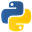```
```// JavaScript -- not that it is for-OF, ****not**** for-IN
const pets = ["dog", "rat", "fish", "rabbit"];
for (const p of pets) {
console.log(p.toUpperCase());
}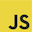```
```# Julia
pets = ["dog", "rat", "fish", "rabbit"]
for p in pets
println(uppercase(p))
end```
```# Perl
my @pets = ("dog", "rat", "fish", "rabbit");
for my \$p (@pets) {
print uc(\$p);
}```
```// Java
var pets = List.of("dog", "rat", "fish", "rabbit");
for (var p: pets) {
System.out.println(p.toUpperCase());
}```
```// C++
vector<string> pets = {"dog", "rat", "fish", "rabbit"};
for (auto p: pets) {
cout << p << '\n';
}```
```// Swift
let pets = ["dog", "rat", "fish", "rabbit"]
for pet in pets {
print(pet.uppercased())
}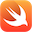```

Oh hey, there’s something cool in Perl...you don’t always need a loop variable—the special variable `\$_` takes on that role. And that variable can even be implicit:

```for (1..10) {print \$_;}
for (1..10) {print}
print for (1..10)```
Hey what about Go and Lua?

Interestingly, we don’t have good examples for iterating through the elements of a sequential collection in Go or Lua. Why not? We’ll see very soon why not.

#### Looping through a collection with indexes

Sometimes you need the index, in addition to the value, within a collection. Some languages offer a function or method, when applied to a collection, to yield Index-Value pairs:

```# Python
pets = ["dog", "rabbit", "rat", "turtle"]
for i, p in enumerate(pets):
print(p, 'is at index', i)```
```// Swift
let pets = ["dog", "rabbit", "rat", "turtle"]
for (i, p) in pets.enumerated() {
print("\(p) is at index \(i)")
}```
```// JavaScript
const pets = ["dog", "rabbit", "rat", "turtle"]
for (let [i, p] of pets.entries()) {
console.log(p, 'is at index', i)
}```
```// Go
func main() {
pets := [...]string{"dog", "rat", "rabbit", "turtle"}
for i, p := range pets {
fmt.Println(p, "is at index", i)
}
}```
```-- Lua
pets = {"dog", "rat", "rabbit", "turtle"}
for i, p in ipairs(pets) do
print(p .. " is at index " .. i)
end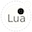```

What’s interesting about Go and Lua is that there is no way to iterate only over the values. To get just the values, you need to explicitly ignore the index! It’s common to do this by naming the index variable `_` and just not touching it.

For-loops are not the only way to do this.

Many languages allow iterating with “each”-functions. We’ll see them very soon.

Of course, there are languages in which you have to use the index only and then grab the value by subscripting:

```-- Ada
for I in 1..10 loop ... A(I) ... end loop;              -- BAD CODE
for I in A'First .. A'Last loop ... A(I) ... end loop;  -- ACCEPTABLE CODE
for I in A'Range loop ... A(I) ... end loop;            -- BEST WE CAN DO```

#### Looping through a dictionary

```# Python
pets = {"Lisichka": "dog", "Clover": "rabbit", "Oreo": "rat"}
for name, kind in pets.items():
print(name, 'is a', kind)```
```// Swift
let pets = ["Lisichka": "dog", "Clover": "rabbit", "Oreo": "rat"]
for (name, kind) in pets {
print("\(name) is a \(kind)")
}```
```// Go
func main() {
pets := map[string]string{"Lisichka": "dog", "Clover": "rabbit", "Oreo": "rat"}
for name, kind := range pets {
fmt.Println(name, "is a", kind)
}
}```
```-- Lua
pets = {Lisichka = "dog", Clover = "rabbit", Oreo = "rat"}
for name, kind in pairs(pets) do
print(name .. " is a " .. kind)
end```
```// JavaScript
const pets = {Lisichka: "dog", Clover: "rabbit", Oreo: "rat"};
for (const [name, kind] of Object.entries(pets)) {
console.log(`\${name} is a \${kind}`);
}```
```// Java
// THIS IS PROBABLY NOT THE BEST WAY.
// SEE THE SECTION ON "EACH" FUNCTIONS BELOW.
var pets = Map.of("Lisichka", "dog", "Clover", "rabbit", "Oreo", "rat");
for (var e: pets.entrySet()) {
System.out.println(e.getKey() + "  is a " + e.getValue());
}```

#### Fancy Loops

In Perl you can dispense with making an array to iterate over and just list the contents of your collection:

```for my \$count (10,9,8,7,6,5,4,3,2,1,"liftoff") {...}
for my \$count (reverse "liftoff", 1..10) {...}```

But here’s something really cool. There’s this neat mix of definite and indefinite iteration in Algol 60, with this cool syntax:

```for i := 1, i+2, while i<10 do ...
```

And something else fancy! Julia can combine nested loops into a single loop:

```for c = 1:40, b = 1:c-1, a = 1:b-1
if a * a + b * b == c * c
println("\$a, \$b, \$c")
end
end```

Finally here’s something boring, and not fancy, but there seems to be no place else to put it. You can use that crazy for-loop syntax in C, Java, C++, and JavaScript to do some interesting things, like iterate through an old-school linked list:

```/* C */
for (int* p = a; p; p = p ->next) ...```

#### Loop Control Modifiers

Many languages give you opportunities to modify loop behavior. You can:

• Completely break out of the whole loop
• `break` (C, Java, Ruby, JavaScript)
• `exit` (Ada, Modula)
• `last` (Perl)
• Immediately start the next iteration (abandon the rest of the current iteration)
• `continue` (C, Java, JavaScript)
• `next` (Perl, Ruby)
• Start the current iteration over
• `redo` (Perl, Ruby)
• Start the entire statement over from the very beginning
• `retry` (Ruby)
Exercise: Fill out this list for many more languages.

Here’s a while loop in Perl using `next`. Perl’s `continue` is something done at the end of every iteration, regardless of how the iteration ended (either normally or through a `next`).

```# Perl
LINE: while (<STDIN>) {
next LINE if /^#/;
next LINE if /^\$/;
# ... process \$_ ....
} continue {
count++;
}```

#### Language Design Considerations for Loops

• Are the bounds evaluated at the beginning of the loop, or can they change during iteration?
• In some languages, the loop variable is read-only. Why?
• In languages that don’t have ways to break out of the middle of a loop, you might get stuck with ugly flags or finding yourself copy-pasting. Then again, there are people that argue that breaking out of a loop prematurely is a hack. Why?
• Should you be allowed to use a `goto` to jump inside an enumeration-controlled loop?
• Sometimes "bounds" are not really bounds! This is an infinite loop in Java (why?):
```for (short i = 30000; i <= 32767; i++) {...}```

### “Each” Functions

This is a very popular alternative to for-loops:

```# Ruby
pets = ["dog", "rat", "fish", "rabbit"]
pets.each {|p| puts p.upcase}```
```// JavaScript
const pets = ["dog", "rat", "fish", "rabbit"];
pets.forEach(p => console.log(p.toUpperCase()));```
```// Java
var pets = List.of("dog", "rat", "fish", "rabbit");
pets.forEach(p -> System.out.println(p.toUpperCase()));```
```// Swift
let pets = ["dog", "rat", "fish", "rabbit"]
pets.forEach {print(\$0.uppercased())}```

Ruby and JavaScript use this technique to produce value and its index during iteration:

```# Ruby
pets = ["dog", "rat", "fish", "rabbit"]
pets.each_with_index {|p, i| puts "#{p} is at index #{i}"}```
```// JavaScript
const pets = ["dog", "rat", "fish", "rabbit"];
pets.forEach((p, i) => console.log("%s is as index %d", p, i));```

Many languages have an each-function for dictionaries, too:

```// Java
var pets = Map.of("Lisichka", "dog", "Clover", "rabbit", "Oreo", "rat");
pets.forEach((name, kind) -> System.out.println(name + " is a " + kind));```
```// JavaScript
const pets = {Lisichka: "dog", Clover: "rabbit", Oreo: "rat"};
Object.entries(pets).forEach(([name, kind]) => {
console.log(`\${name} is a \${kind}`);
});```
```# Ruby
pets = {Lisichka: "dog", Clover: "rabbit", Oreo: "rat"}
pets.each {|name, kind| puts "#{name} is a #{kind}"}```

Many languages use `each` to build up dozens of methods that work on each item of a collection. Examples include `map`, `filter`, `every`, `some`, `min`, and `max`. The effect of all this is that you might find yourself writing few, if any, loops when processing collections!

### Iterator Objects

An iterator is an object that keeps track of where you are during an iteration. You don’t have to use them in a loop! You just call methods such as `hasNext` and `next` (or in some languages, `begin`, `end`, and `++`).

```// Java - variable types shown explicitly for emphasis
List<String> pets = Arrays.asList("dog", "rat", "rabbit");
Iterator<String> it = pets.iterator();
System.out.println(it.hasNext());               // true
System.out.println(it.next());                  // dog
System.out.println(it.hasNext());               // true
System.out.println(it.next());                  // rat
System.out.println(it.hasNext());               // true
System.out.println(it.next());                  // rabbit
System.out.println(it.hasNext());               // false
System.out.println(it.next());                  // raises NoSuchElementException```
```// C++
vector<string> pets = {"dog", "rat"};
vector<string>::iterator it = pets.begin();
cout << *it << '\n';                            // dog
it++;
cout << *it << '\n';                            // rat
it++;
cout << (it == pets.end());                     // 1```
Exercise: What happens if you access `*it` when `it` is at the end? What if you try `it++`?
```# Python
pets = ["dog", "rat"]
it = iter(pets)
next(it)                     # "dog"
next(it)                     # "rat"
next(it)                     # raises a StopIteration exception!```
```# Python
def colors():
yield 'red'
yield 'green'
yield 'blue'

it = colors()
next(c)                      # "red"
next(c)                      # "green"
next(c)                      # "blue"
next(c)                      # raises a StopIteration exception!```

The use of explicit iterators opens the possibility that your underlying collection changes while your iterator is still active. In some languages, this may cause your program to just crash; in others, doing this will trigger a "concurrent modification exception."

```// Java
var it = pets.iterator();
System.out.println(it.next());                  // dog
System.out.println(it.next());                  // raises ConcurrentModificationException```
Exercise: Write a paper, with code snippets, about iterator objects in C++, Python, and Ruby.

## Recursion

A recursive subroutines calls itself. Recursion is

• As powerful as iteration
• Often more natural than iteration when doing functional programming
• Often less natural than iteration when doing imperative programming
• Not allowed in some languages (e.g., pre-1990 Fortran)

Recursive code is

• Usually shorter than its iterative equivalent
• Usually easier to prove correct than its iterative equivalent
• Sometimes easy to misuse, resulting in horrid code

What could go wrong?

```(* THIS IS A REALLY STUPID USE OF RECURSION *)
fun fact n = if n < 0 then 1 else n * fact(n-1)
```

evaluates as

```fact 4
= 4 * fact 3
= 4 * (3 * fact 2)
= 4 * (3 * (2 * fact 1))
= 4 * (3 * (2 * (1 * fact 0)))
= 4 * (3 * (2 * (1 * 1)))
= 4 * (3 * (2 * 1))
= 4 * 3 * 2
= 4 * 6
= 24
```

Here, all these pieces of partially complete computations take up a lot of space, hurting performance.

### Tail Recursion

Tail recursive code can always be implemented efficiently. Contrast that last factorial implementation with this one:

```(* ML, Tail Recursive *)
fun gcd x y = if y = 0 then x else gcd y (x mod y)```
```-- Haskell, Tail Recursive
gcd x y = if y == 0 then x else gcd y (x `mod` y)```
```// JavaScript, Tail Recursive
const gcd = (x, y) => y === 0 ? x : gcd(y, x % y);```

This evaluation is much cleaner:

```gcd 444 93
= gcd 93 72
= gcd 72 21
= gcd 21 9
= gcd 9 3
= gcd 3 0
= 3
```

A tail recursive function returns exactly the result of calling itself — no partial results need to be stored. A good compiler can recognize tail recursion and generate code that has no calls at all: just reload the parameters and jump back to the top. It’s as if you wrote:

```# Ruby
def gcd(x, y)
while true
return x if y == 0
x, y = y, x % y
end
end```

though the tail-recursive code is cleaner, and better because it has no side effects!.

In functional programming the programmer will sometimes have to turn a non-tail-recursive function into a tail recursive one. The idea is to "pass along" arguments (like a counter or partial result) "into" the next call. So factorial can be written

```(* ML *)
fun fact n =
let
fun f i a = if i = n then a else f (i+1) ((i+1)*a)
in
f 0 1
end```

which is evaluated like so

```fact 5
= f 0 1
= f 1 1
= f 2 2
= f 3 6
= f 4 24
= f 5 120
= 120
```

and Fibonacci like this

```(* ML *)
fun fib n =
let fun f i last current =
if i = n then current else f (i+1) current (last+current)
in
f 0 0 1
end```

This yields

```fib 7
= f 0 0 1
= f 1 1 1
= f 2 1 2
= f 3 2 3
= f 4 3 5
= f 5 5 8
= f 6 8 13
= f 7 13 21
= 21
```

This sure beats the naive implementation, which requires 535,821,591 calls to compute fib(40).

Exercise: Oops, these functions don’t handle an initial negative n. Fix them.

## Nondeterminacy

Nondeterministic control flow occurs when the next computation step is made randomly (not arbitrarily) from a set of alternatives, something like:

```select
x := 4;
or
y := 6;
or
print "Hello";
end;
```

Generally each of the arms are guarded. To execute a select statement, first the guards are evaluated, then a choice is made among the open alternatives (those with true guards). A missing guard is assumed to be true. Example

```select when x > y =>
y := x * x - 3;
print x;
or
close(f);
or when x % y >= 25 || finished =>
crash();
end;
```

What if all guards are false? In Ada, this raises an exception. In other languages, the statement simply has no effect.

Nondeterministic control flow can hide some asymmetries in code. Some examples:

```select when (a >= b) =>
max := a;
or when (a <= b) =>
max := b;
end;
```
```loop
select when a > b =>
a := a - b;
or when b > a =>
b := b - a;
or when a = b =>
return a;
end;
end;
```

Nondeterministic constructs turn out to be very useful in concurrent programming, because random execution paths sometimes help to avoid deadlock.

## Concurrency

This is a huge topic. So it is covered separately.

## Summary

We’ve covered:

• Kinds of control flow
• Declarations, Expressions, and Statements
• Sequencing
• Selection (if, switch, case)
• Iteration (while, repeat, for, iterator objects)
• Recursion
• Tail recursion
• Non-determinacy